: 3 Turbulent parameterization : Two dimensional anelastic model : 1 Outline of the

# 2 Atmospheric model

The governing equation of model atmospheric dynamics is the 2D anelastic system (Ogura and Phillips, 1962).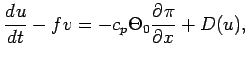(1)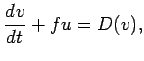(2)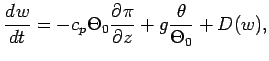(3)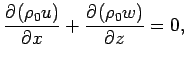(4)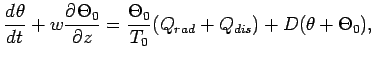(5)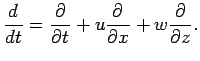(1), (2), (3) are the horizontal and vertical component of equation of motion, respectively. (4) is the continuity equation and (5) is the thermodynamic equation.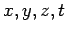are horizontal, vertical and time coordinate, respectively.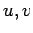are horizontal and vertical velocity, and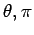are potential temperature and nondimensional pressure function deviation from those of basic state, respectively.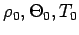are density, potential temperature and temperature in basic state.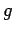is gravitational acceleration whose value is equal to 3.72 msec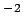.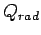is radiative heating (cooling) rate per unit mass which is calculated by convergence of the radiative heat flux.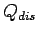is heating rate per unit mass owing to dissipation of turbulent kinetic energy, which is given by turbulent parameterization.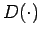term in equation (1)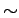(5) represents the turbulent diffusion owing to subgrid scale turbulent mixing.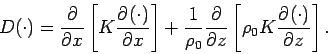(6)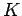is turbulent diffusion coefficient which is calculated by (10) and (11).

The nondimensional pressure function and potential temperature are defined as follows.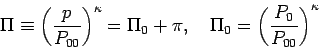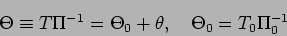where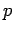and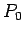are pressure and basic state pressure,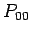is reference pressure (= 7 hPa),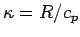,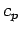is specific heat of constant pressure per unit mass and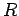is atmospheric gas constant per unit mass. The basic state atmospheric structure is calculated by using the hydrostatic equation as follows.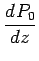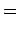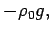(7)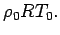(8)

The deviation of nondimensional pressure function is calculated by using the following equation which is derived from (1)(4).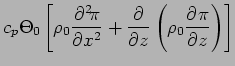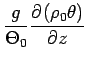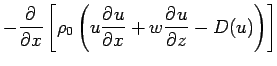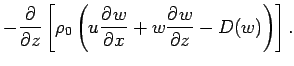(9)

## Boundary conditions

The model horizontal boundary is cyclic. The vertical wind velocity is set to be 0 at the surface and upper boundary.

## Parameters

 Parameters Standard Values Note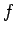0 sec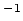3.72 msec7 hPa734.9 JkgKValue of CO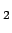189.0 JkgKValue of CO: 3 Turbulent parameterization : Two dimensional anelastic model : 1 Outline of the
Odaka Masatsugu $BJ?@.(B19$BG/(B4$B7n(B25$BF|(B